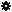﻿ Object Model: EdgeCollection.NeighborPolygons

EdgeCollection.NeighborPolygons

## EdgeCollection.NeighborPolygonsv3.0

### Description

Return a collection of PolygonFace objects for all neighbors within a given distance.

### C# Syntax

 ```PolygonFaceCollection EdgeCollection.NeighborPolygons( Int32 in_lDistance ); ```

### Scripting Syntax

 ```oReturn = EdgeCollection.NeighborPolygons( [Distance] ); ```

### Parameters

Parameter Type Description
Distance Integer Distance is an integer value representing the degree of neighborhood (eg: degree=2 for a polygon means its adjacent polygons plus the adjacent polygons of the adjacent polygons).

Default Value: 1

### Examples

#### VBScript Example

 ```set oCube = ActiveSceneRoot.AddGeometry( "Cube", "MeshSurface" ) set oGeometry = oCube.ActivePrimitive.Geometry set oPolygons = oGeometry.Polygons set oNeighborEdges = oPolygons(0).NeighborEdges(1) set oNeighborPolygons = oNeighborEdges.NeighborPolygons( 1 ) str = "Polygons neighbors of this EdgeCollection are :" for each n in oNeighborPolygons str = str & " " & n.index next logmessage str ```